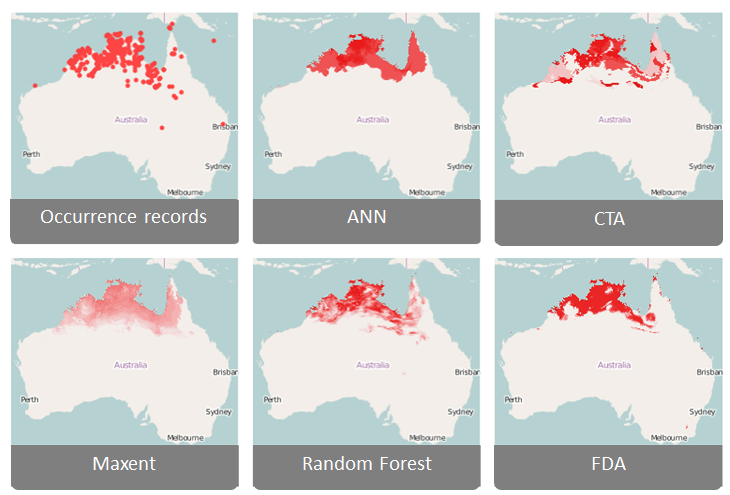It is important to understand how to interpret the output of a model. A vital step in modelling is assessing the accuracy of the model prediction, commonly called ‘validation’ or ‘evaluation’. There is a variety of different outputs that your species distribution model will produce, and that you can evaluate to decide whether your model is valid. While the focus in model evaluation is often on the predictive performance of the model, which can be measured by a variety of evaluation statistics, it is important to also do a reality check of the visual outputs such as the predicted distribution map and the response curves of the environmental variables.

## Step 1: Critically evaluating the predicted distribution of your species.

During the design phase of your project, we suggested you do a bit of research on the species before designing your species distribution model, so you should have some idea of what the likely distribution of the species is. When you look at the map produced by the species distribution model you should be able to critically evaluate whether the predicted distribution is plausible, while taking into account factors such as dispersal barriers.  For example, if you look at this map with the predicted distribution of grey-headed flying fox, we see that this species is predicted to be present in some areas in Western Australia, while the species has never been observed there. So, while the conditions in this area might be suitable for the species to survive, there could be natural barriers that prevent the species from dispersing there.## Step 2: Critically evaluating the response curves for each environmental variable.

Response curves show the probability of occurrence for each value of the environmental variable, while taking into account the other variables that were put into the model. With the knowledge that you have about the species, check whether these response curves conform to the known tolerances of the environmental conditions. For example, if the species is known to not survive above certain temperatures, you expect this to be reflected in the response curve.The predictive performance of the model can be assessed with a suite of quantitative measures, known as evaluation statistics. To obtain these statistics, first calibrate the model with a set of training data, and then validate the model with a set of test data. This validation is best done with a set of test data that is independent of the training data. This means that this should be data that is not used to fit the model. A common approach that is used to achieve this is called cross-validation.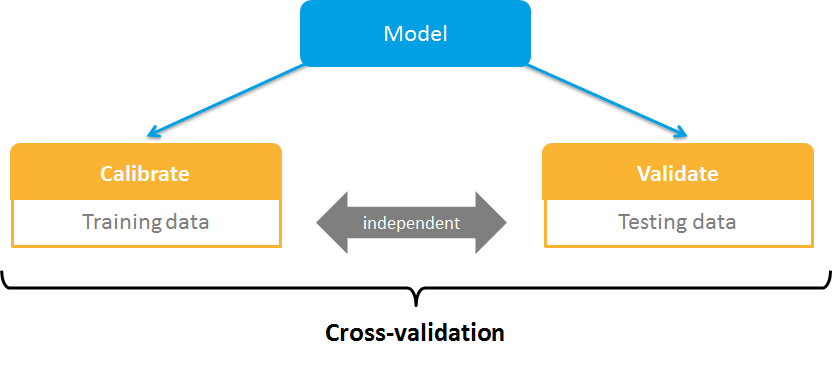## Step 3: Cross Validation

In cross-validation the total dataset, which means all your records of presences and absences of a species, is divided into a predefined number of subsets, also called folds. The model is calibrated with all but one of the folds, and the fold that is not used in calibration is used for validation.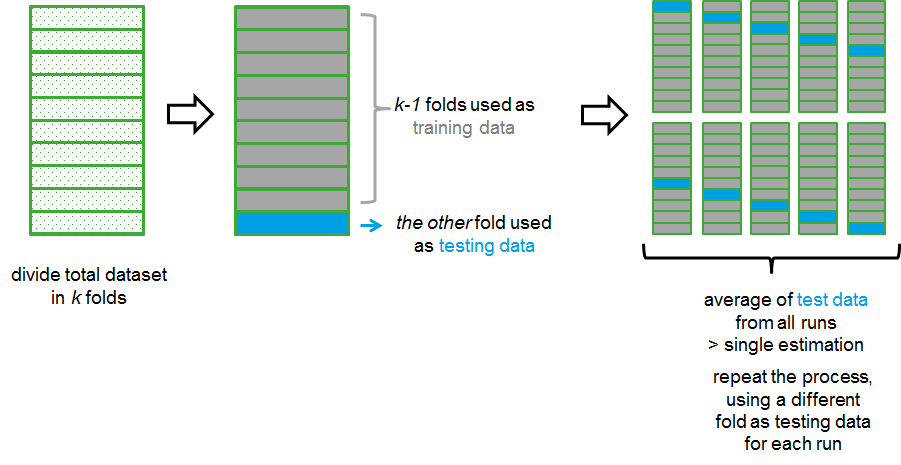This process is repeated as many times as the number of folds, so in this example 10 times, and each of the folds is used once as the testing dataset. The results obtained with the testing data from all 10 runs are then averaged to produce a single estimation. 10-fold cross validation as in this example is commonly used, and often the default in packages that run species distribution models, but in theory you can use any number of folds such as 3-fold or 5-fold. The extreme version is the leave-one-out cross-validation approach, in which the process is repeated as many times as there are data points, and for each run only 1 data point is left out to train the model, and that one point is used for validation.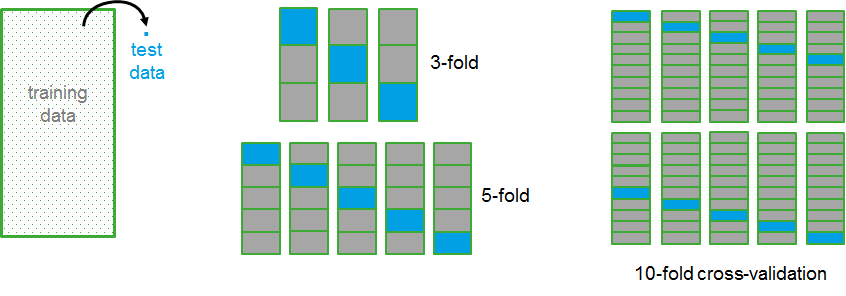The cross-validation approach averages the results of the test data of the different runs into a  single probability score of species occurrence for each location on the map.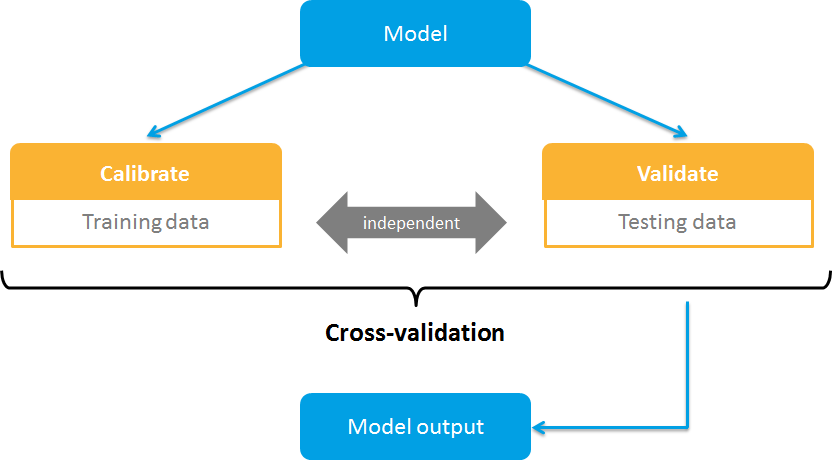## Step 4: Categorical Prediction

Most algorithms produce this probability score as a continuous response ranging from 0, which represents a low probability of presence, to 1, which represents a high probability of presence. To calculate the evaluation statistics, these probabilistic predictions are commonly converted to a categorical prediction, which means that for any given point it is predicted whether a species could be present or absent. This conversion is based on a threshold value of the probability prediction. By convention, this threshold is often set at 0.5, which means that for each location with a probability above 0.5, the prediction is positive, thus the species is present, and locations with a probability below 0.5 are predicted to be negative, thus the species is absent. However, there are a lot of different methods for selecting the threshold value depending on factors such as the overall error pattern of the model and the ratio of presence vs absence points.The categorical predictions of a species distribution model, thus whether a species is present or absent in a particular site, can either be correct or incorrect. The predictions are compared to the actual observations, and a correct prediction is referred to as a true positive for presences and a true negative for absences. The two different types of errors that can be made are a ‘false positive’ when the model predicts a species to be present in places where it has not been observed, or a ‘false negative’ means that the model predicted a species to be absent in places where it is observed to be present. A table in which the performance of a model is summarized like this is called a contingency table.## Step 5: Accuracy and Misclassification Rate

A simple measure of the predictive performance of a model is Accuracy, which simply measures the proportion of correctly predicted cases by summing the true positives and true negatives and divide this sum by the total count. The opposite function of Accuracy is the Misclassification Rate, which sums all the false positives and false negatives and divides this by the total count.

Although these measures are easy to understand and interpret, they don’t distinguish between the two error types, false positives and false negatives. Additionally, they don’t take into account the proportion of presence records relative to the absence records. To illustrate this with an extreme example, if you were modelling the distribution of a rare species with a low number of observed presences, the model can have an accuracy of 0.9 by just predicting all sites to be absent. This corresponds to a misclassification rate of 0.1, thus only 10% of all records were predicted incorrectly, but the prediction of zero presences is obviously not correct. Therefore, other evaluation statistics that are used more often are the True and False Positive Rate, and the True and False Negative Rate.## Step 6: Sensitivity and Specificity

The True Positive Rate refers to the proportion of observed presences that are correctly predicted. This is calculated as the number of true positives divided by the sum of true positives and false negatives. The True Positive Rate is often named Sensitivity. The opposite of the True Positive Rate is the False Negative Rate. A high True Positive Rate indicates a good performance of the model. Like in this example, the True Positive Rate is 0.9, which automatically means that the False Negative Rate is 0.1. This means that 90% of the observed presences are correctly predicted as being present. Note that these two statistics can be calculated if you only have presence data.The True Negative Rate refers to the proportion of observed absences that are correctly predicted. This is calculated as the number of true negatives divided by the sum of false positives and true negatives. The True Negative Rate is also referred to as Specificity.

The opposite of the True Negative Rate is the False Positive Rate. Again, a high True Negative Rate indicates a good performance of the model. If we look again at the example, we find a True Negative Rate of 0.96, and a False Positive Rate of 0.04, indicating that 96% of the observed absences are correctly predicted. You can check whether this prediction is statistical significant by testing whether the True Positive or Negative Rates are higher than would be expected by chance.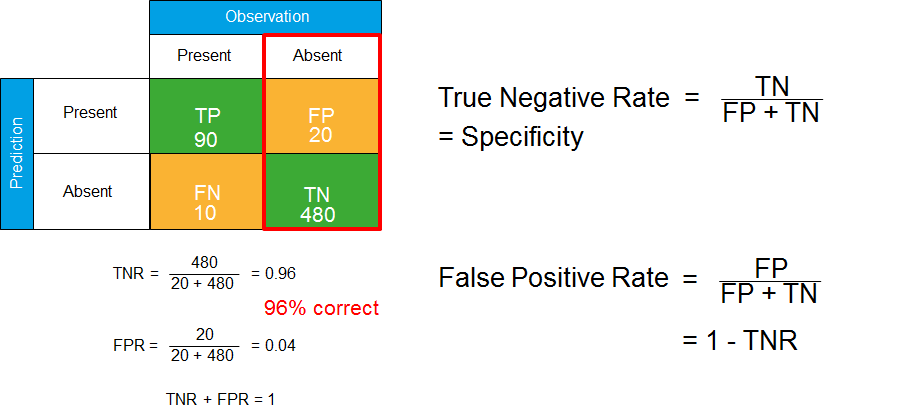Because the elements of the contingency table are dependent on the value of the probability threshold, the threshold value regulates the outcomes of the evaluation statistics. With an increasing threshold value, the number of predicted presences will decrease. The number of observed presences remains the same, and thus the proportion of presences that is correctly predicted will decrease. The below graph shows the threshold value on the x-axis, and the value of the True Positive and True Negative Rate on the y-axis. With an increasing threshold value, the model will predict more absences and less presences, and thus the number of observed presences that are correctly predicted will decrease. This means that the True Positive Rate decreases with an increasing threshold value, while the True Negative Rate increases.## Step 7: How do you select the threshold value to evaluate your model?

There are a number of different methods that you can use to select the threshold value in your model, below we highlight a few of these. A common default method is to stick with a value of 0.5, but this is often not appropriate. It works well when the data has an even number of presences and absences, but not always, since the model needs to predict absences and presences equally well. In this example, at the value of 0.5 the True Positive Rate is only 0.12, meaning that only 12% of the observed presences were correctly predicted. Another method is to choose for a fixed True Positive Rate, such as 95%, which corresponds here to a low threshold value. But this option automatically results in a low True Negative Rate, and thus a high False Positive Rate. Therefore, other methods that trade off the successful predictions and errors are more often used. For example, selecting the threshold where the True Positive Rate is equal to the True Negative Rate. This is the point in the graph where the two lines cross over. You can also choose the value where the sum of the True Positive and the True Negative rate is maximized.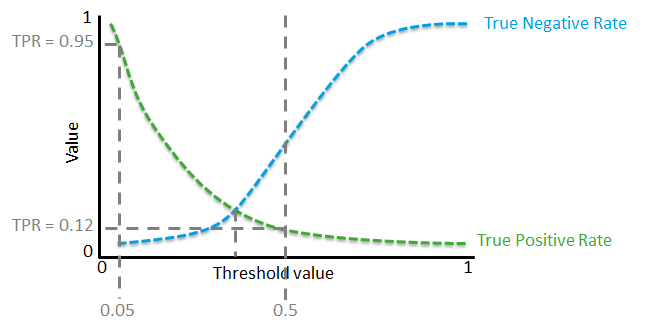## Step 8: Relative Operating Characteristic (ROC)

One performance measure that is also commonly used, and not dependent of the threshold probability value is the Relative Operating Characteristic or ROC plot. The ROC plot is a graph with the False Positive Rate on the x-axis and the True Positive Rate on the y-axis plotted across the range of possible thresholds. A perfect model would only include true positives and no false positives, displayed by the dot in this graph, representing a false positive rate of 0, and a true positive rate of 1. The curve across all possible thresholds would look like this. A random guess of the model would result  in a point along the diagonal line from the left bottom to the right corner. This is the divider of the ROC space. Any point above the line represents predictions that are better than random, whereas points below the line represent poor predictions. The value for ROC is the area under the curve (AUC), and is calculated by summing the area under the ROC curve. A value of 0.5 thus represents a random prediction, and values above 0.5 indicate predictions better than random. The closer the ROC curve follows the y-axis, the larger the area under the curve, and thus the more accurate the model. In general, AUC values of 0.5–0.7 are considered low and represent poor model performance, values between 0.7 and 0.9 are considered moderate, and values above 0.9 represent excellent model performance.Like with all aspects of species distribution models, the selection of the evaluation statistics that you use to evaluate your model depends on various factors. The evaluation statistics that are available depend on the type of data that you use in your model. Some statistics need both presence and absence data to be calculated, and can thus not be used if you only use presence data. Also keep in mind, your research question, or how you are going to apply the outcomes of the model. Some statistics are better to evaluate predictions of the actual distribution of a species, whereas others might be more useful If you are interested in the potential distribution, for example when you study an invasive species.

## Step 9: Comparing multiple algorithm outputs

You may also want to compare model outputs and evaluation statistics across various algorithms to get a better insight into which algorithm has performed better with your data.